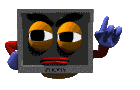Formatting Output

#include <iostream.h>
#include <iomanip.h>

Formatting output is important in developing output screens which can be easily read and understood by the program users.  C++ offers the programmer several input/output manipulators. Two of these I/O manipulators are setw and setprecisionThe setw manipulator sets the width of the field assigned for the output.  It takes the size of the field (in number of characters) as a parameter.

For example, the code:

cout << setw(6) <<"R";

generates the following output on the screen (each underscore represents a blank space)

_ _ _ _ _R

The setw( ) is the only manipulator that does not stick from one command to the next.  If you want to right-justify three numbers with an 8-space output width, you will need to repeat setw( ) in this manner:
cout << setw(8) << 22 << "\n";
cout << setw(8) << 4444 << "\n";
cout << setw(8) << 666666 << endl;

The output will be (each underscore represents a blank space)
_ _ _ _ _ _ 2 2
_ _ _ _ 4 4 4 4
_ _ 6 6 6 6 6 6The setprecision manipulator sets the total number of digits to be displayed when floating point numbers are output.

For example, the code:

cout << setprecision(5) << 123.456;

generates the following output on the screen (notice the rounding):

123.46

The setprecision manipulator can also set the number of decimal places displayed.  If you wish to use setprecision in this manner, you must set an ios flag.  The flag is set with the following statement:

cout.setf(ios::fixed);

Once the flag has been set, the number you pass as a parameter to setprecision is the number of decimal places you wish displayed.  For example, the code:

cout.setf(ios::fixed);
cout << setprecision(5) << 12.345678;

generates the following output on the screen (notice no rounding):

12.34567Additional IOS flags: cout.setf(ios::fixed) // "fixed" is a format option

Other format options include:

 left left-justifies the output right right-justifies the output showpoint displays decimal point and trailing zeros for all floating point numbers, even if the decimal places are not needed. uppercase displays the "e" in E-notation as "E" rather than "e" showpos displays a leading plus sign before positive values scientific displays floating point numbers in scientific ("E") notation fixed displays floating point numbers in normal notation - no trailing zeroes and no scientific notation

**Note: If neither the scientific nor fixed option is set, the compiler decides upon the method used to display floating-point numbers based on whether the number can be displayed more efficiently in scientific or fixed notation.

**Note: You can remove these options by replacing setf with unsetf.

Example:

#include <iostream.h>

int main(void)
{
float x = 18.0;
cout<< x << endl;               //displays 18
cout.setf(ios::showpoint);
cout<< x << endl;               //displays 18.0000
cout.setf(ios::scientific);
cout<< x << endl;               //displays 1.800000e+001
cout.unsetf(ios::showpoint);
cout.unsetf(ios::scientific);
cout<<x<<endl;                  //displays 18

return 0;
}

To print 5.8 as 5.80 the following lines of code are needed
(particularly nice for displaying amounts of money):

cout.setf(ios::fixed);              //Sets the cout flag for fixed-point
//non-scientific notation trailing zeros

cout.setf(ios::showpoint);    //Always shows decimal point
cout << setprecision(2);      //Two decimal places
cout << 5.8;

 All subsequent couts retain the precision set with the last setprecision( ). Think of setprecision as being "sticky." Whatever precision you set sticks with the cout device until you change it with a subsequent setprecision( ) later in the program.